# ISEE Upper Level Quantitative : Radius

## Example Questions

### Example Question #3 : How To Find The Area Of A Circle

Compare the two quantities:

Quantity A: The area of a circle with radiusQuantity B: The circumference of a circle with radiusThe relationship cannot be determined from the information given.

The quantity in Column B is greater.

The two quantities are equal.

The quantity in Column A is greater.

The quantity in Column A is greater.

Explanation:

Recall for this question that the formulae for the area and circumference of a circle are, respectively: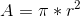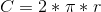For our two quantities, we have:

Quantity A: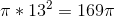Quantity B: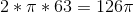Therefore, quantity A is greater.

### Example Question #11 : Radius

The radius of a circle is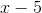. Give the area of the circle in terms of.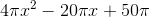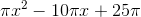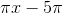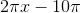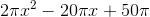Explanation:

The area of a circle with radiuscan be found using the formulaSince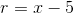, the area is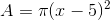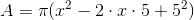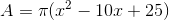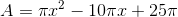### Example Question #1 : How To Find The Area Of A Circle

The radius of a circle is. Give the circumference of the circle in terms of.Explanation:

The circumference of a circle istimes its radius. Therefore, since the radius is, the circumference is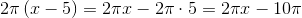### Example Question #6 : How To Find The Area Of A Circle

The areas of six circles form an arithmetic sequence. The second-smallest circle has a radius twice that of the smallest circle.

Which is the greater quantity?

(a) The area of the largest circle.

(b) Twice the area of the third-largest circle.

It is impossible to tell which is greater from the information given

(a) is greater

(b) is greater

(a) and (b) are equal

(b) is greater

Explanation:

Letbe the radius of the smallest circle. Then the second-smallest circle has radius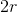. Their areas, respectively, are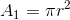and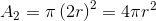The areas form an arithmetic sequence, so their common difference is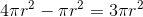.

The six areas are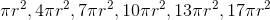The third-largest circle has area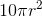; twice this is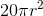. This is greater than the area of the largest circle, which is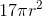. (b) is the greater quantity.

### Example Question #7 : How To Find The Area Of A Circle

The radii of six circles form an arithmetic sequence. The radius of the second-smallest circle is twice that of the smallest circle. Which of the following, if either, is the greater quantity?

(a) The area of the largest circle

(b) Twice the area of the third-smallest circle

It is impossible to tell which is the greater from the information given

(a) is greater

(a) and (b) are equal

(b) is greater

(a) is greater

Explanation:

Call the radius of the smallest circle. The radius of the second-smallest circle is then, and the common difference of the radii is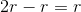.

The radii of the six circles are, from least to greatest: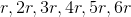The largest circle has area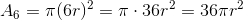The third-smallest circle has area: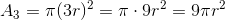Twice this is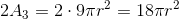The area of the sixth circle is greater than twice that of the third-smallest circle, so the correct choice is that (a) is greater.

### Example Question #8 : How To Find The Area Of A Circle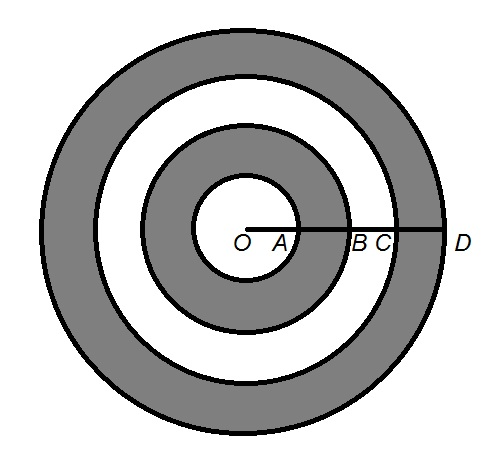In the above figure,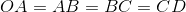.

Which is the greater quantity?

(a) Twice the area of inner gray ring

(b) The area of the white ring

(a) is the greater quantity

It is impossible to determine which is greater from the information given

(a) and (b) are equal

(b) is the greater quantity

(a) is the greater quantity

Explanation:

For the sake of simplicity, we will assume that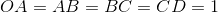; this reasoning is independent of the actual length.

The four concentric circles have radii 1, 2, 3, and 4, respectively, and their areas can be found by substituting each radius forin the formula: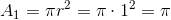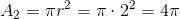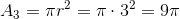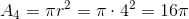The white ring has as its area the difference of the areas of the second-largest and third-largest circles: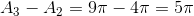The inner gray ring has as its area the difference of the areas of the third-largest and smallest circles: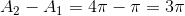.

Twice this is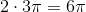, which is greater than the area of the white ring.

### Example Question #11 : RadiusIn the above figure,.

Which is the greater quantity?

(a) Six times the area of the white circle

(b) The area of the outer ring

It is impossible to determine which is greater from the information given

(a) is the greater quantity

(b) is the greater quantity

(a) and (b) are equal

(b) is the greater quantity

Explanation:

For the sake of simplicity, we will assume that; this reasoning is independent of the actual length.

The four concentric circles have radii 1, 2, 3, and 4, respectively, and their areas can be found by substituting each radius forin the formula:The outer gray ring is the region between the largest and second-largest circles, and has area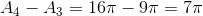Six times the area of the white (inner) circle is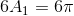, which is less than the area of the outer ring,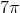.

### Example Question #1 : How To Find Circumference

A giant clock has a minute hand five yards in length. Since noon, the tip of the minute hand has traveled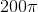feet. Which is the greater quantity?

(A) The amount of time that has passed since noon

(B) The amount of time until midnight

(B) is greater

It is impossible to determine which is greater from the information given

(A) and (B) are equal

(A) is greater

(A) is greater

Explanation:

Five yards is equal to fifteen feet, which is the length of the minute hand. Subsequently, fifteen feet is the radius of the circle traveled by its tip in one hour; the circumference of this circle istimes this, or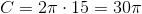feet.

In one six-hour period, the minute hand revolves six times, so its tip travles six times the circumference, or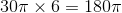The clock has traveled farther than this, so the time is later than 6:00 PM, and more time has elapsed since noon than is left until midnight. This makes (A) greater.

### Example Question #1 : How To Find Circumference

Compare the two quantities:

Quantity A: The area of a circle with radiusQuantity B: The circumference of a circle with radius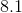The quantity in Column A is greater.

The quantity in Column B is greater.

The relationship cannot be determined from the information given.

The two quantities are equal.

The quantity in Column A is greater.

Explanation:

Recall for this question that the formulae for the area and circumference of a circle are, respectively:For our two quantities, we have:

Quantity A: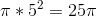Quantity B: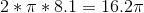Therefore, quantity A is greater.

### Example Question #3 : How To Find Circumference

The circumferences of eight circles form an arithmetic sequence. The smallest circle has radius two inches; the second smallest circle has radius five inches. Give the radius of the largest circle.

2 feet

3 feet 10 inches

4 feet 2 inches

2 feet, 1 inch

1 foot, 11 inches

1 foot, 11 inches

Explanation:

The circumference of a circle can be determined by multiplying its radius by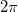, so the circumferences of the two smallest circles are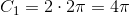and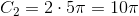The circumferences form an arithmetic sequence with common difference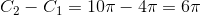The circumference of a circle can therefore be found using the formula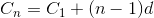where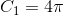and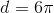; we are looking for that of the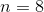th smallest circle, so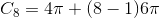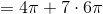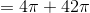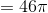Since the radius of a circle is the circumference of the circle divided by, the radius of this eighth circle is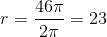inches, or 1 foot 11 inches.

### All ISEE Upper Level Quantitative Resources